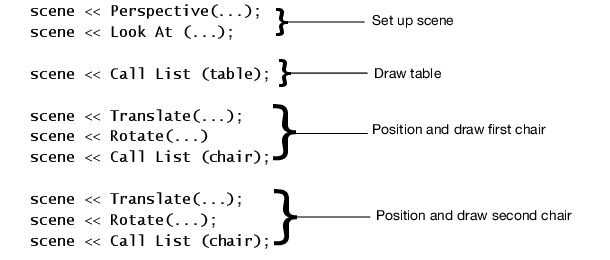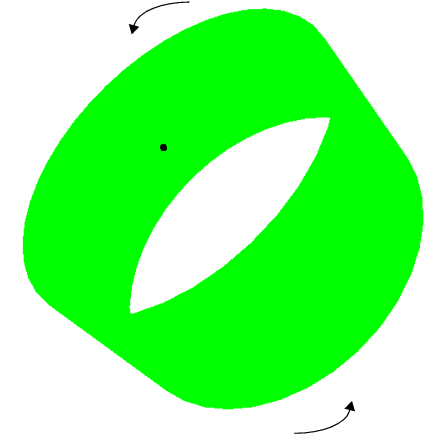Publication date: 05/24/2021

## The Rotate Command

The Rotate command is used to modify the viewing angle of a scene. It has the following format.

`Rotate (degrees, xAxis, yAxis, zAxis)`

This rotates by degrees around the axis described by the vector (xAxis, yAxis, zAxis). For example, to rotate a model 90 degrees about the x-axis, use Rotate( 90, 1, 0, 0 ).

You can also specify the three axis values in a matrix. For example, Rotate( 90, [1, 0, 0] ).

Note: The Rotate command uses degrees, in contrast to JMP’s trigonometric functions, which use radians.

Translate and Rotate are also used to position objects with respect to each other. The first Translate or Rotate can be thought of as positioning everything that follows with respect to the camera. Subsequent Translate and Rotate commands are used to position objects, such as spheres, cylinders, disks, and display lists in Call List and ArcBall commands. For example, suppose you have a display list named table and another named chair. Your scene might look like this:

Figure 13.7 Using Translate and RotateThe following example uses the Rotate command inside a For loop to continuously change the viewing angle of a scene. It draws a cylinder that swings around a central point. This central point is shown by a small sphere.

`scene = Scene Box( 600, 600 ); // make a scene box...holds an OpenGL scene.`
` `
`New Window( "Example 1", scene ); // put the scene in a window`
` `
`For( i = 1, i < 360, i++,`
`	scene << Clear;`
` `

// the lens is 50 degrees, near is 1 units from the camera, far is 10.

`	scene << Perspective( 50, 1, 10 );`
`	scene << Translate( 0.0, 0.0, -2 );`
` `
`	scene << Rotate( i, 1, 0, 0 );`
`	scene << Rotate( i * 3, 0, 1, 0 );`
`	scene << Rotate( i * 3 / 2, 0, 0, 1 );`
`	scene << Color( 0, 1, 0 ); // green for cylinder`
`	scene << Cylinder( 0.5, 0.5, 0.5, 40, 10 );`
`	scene << Color( 0, 0, 0 ); // black for sphere`
`	scene << Sphere( 0.01, 10, 5 );`
`	scene << Update;`
`	Wait( 0.01 ); );`

Figure 13.8 Rotating a CylinderNote the use of the Update command at the end of the scene messages. This command tells JMP to make the displayed screen agree with the current state of the display list. It is important to clear the list at the beginning (so that the list does not contain the old angles as well as the current) and update the scene after each change.